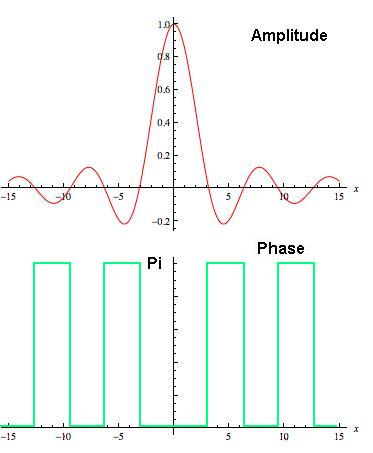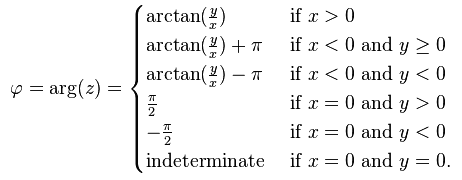# Diffraction - Amplitude and Phase

Hello,
I'm trying to understand how can I compute a phase graph from diffraction. I will follow here Fourier transform formalism of finding the light distribution on screen after diffraction.
I'm considering a 1D problem. Suppose I have a single slit of width a. This slit can be mathematically written as:
$$y=Arect\left ( \frac{x}{a} \right )\bigotimes \delta \left ( 0 \right )$$
i.e it is some amplitude A, multiplied by a rectangular function of width "a" with a convolution with delta function, since it's at origin.
Diffraction pattern is given by a Fourier transform:
$$Y=Aasinc\left ( \pi ka \right )$$
So, the amplitude of the resulting diffraction pattern is given above (roughly ~sinc function). Spatial friquency k is:
$$k=\frac{x}{\lambda D}$$
Where x is a position on the screen, D is the distance from the slit to the screen and lambda is a wavelength.
The intensity distribution on the screen is then:
$$I=\left |Y \right |^2=A^2a^2sinc^2\left ( \pi ka \right )$$

Till now everything is fine. But in order to get the slit image, i.e. performing an inverse Fourier transform, I need both amplitude and phase. Amplitude I have calculated above (~sinc), but what is a phase as a function of x (position on the screen)? Is it zero everywhere? I know that phase in this case will be zero at point where amplitude is positive and pi at points where amplitude is negave:But how do I see the phase vs x from the diffraction pattern distribution?

Thanks!

blue_leaf77
Homework Helper
how do I see the phase
Do you mean see visually? If yes, you can't, our eyes are not made sensitive to the phase of light.

•LmdL
marcusl
Gold Member
When you take the absolute value squared to find intensity, you lose all phase information. (In other contexts this quantity is called a power spectrum, and power, like intensity, is purely real and non-negative.) As a result, you cannot recover the aperture function with an inverse Fourier transform (IFT).

If you take the IFT of the intensity, you get the spatial autocorrelation function of the aperture. This operation is called the Wiener-Khinchin theorem. For some simple cases, the aperture can be deduced through a process called deconvolution. In the present case, the autocorrelation function is a triangle that is twice the width of the rectangular aperture, and it is known that a triangle results from the convolution of a rectangle with itself (see a book of Fourier transforms), so you can get to it that way. This isn't easy for complicated aperture functions.

•LmdL
Thanks guys! I already found the answer myself, which is: in the above case, Fourier transform is real (without imaginary part), so if I want to represent the amplitude on the screen I will write:
$$Y=Aasinc\left ( \pi ka \right )=Re^{i \phi}$$

since the transform is real, Φ can be either zero or pi. To know if its zero or pi for a particular point I represent the amplitude as complex number Y=x+iy
So in this case:
$$x=Aasinc\left ( \pi ka \right ),y=0$$

The phase is the argument of the complex number and is given by (from wiki):So in my case y=0 always (no complex part), thus when my sinc function is positive (x positive) - phase is 0, and when it's negative (x negative) - phase is pi.
Thank you both anyway!

marcusl